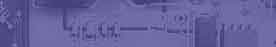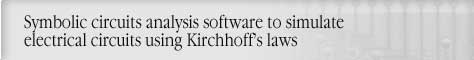Kirchhoff's laws,1- Kirchhoffs Voltage Law,2 - Kirchhoffs Current LawCircuit analysis Tutorial
AKNM Circuit Magic- circuit analysis software

Kirchhoff's Current Law (KCL)

KCL states that the algebraic sum of the currents in all the branches which converge in a common node is equal to zero

SIin = SIout

Kirchhoff's Voltage Law

Kirchhoff's Voltage Law states that the algebraic sum of the voltages between successive nodes in a closed path in the network is equal to zero.

SE = SIR

Solution using KirchhoffÆs Voltage and current laws

Steps to solve circuit by KirchhoffÆs Laws.

1. Construct circuit with circuit magic schematics editor.Circuit sample from circuit magic.

2. Construct loops. (See ôcreating loopö section in user guide) Number of loops (and number of KirhhoffÆs Voltage laws equations) can be determined using following formula. Loop can not include branches with current sources. Due current sources resistance equal infinity.

Loop Number = Branch Number û(Nodes Number û1) û Current sources Number

3. Select Analyze->Solve by KirhhoffÆs laws menu item

1. In dialog box press OK button. if no warning shown.

Solution example from circuit magic.

Writing Kirchhoff current law for 3-1 nodes

(Note number of Kirchhoff current laws equations equal Nodes Number û1)

(Node 1)J1+I3+I4+I7=0

(Node 2)-J1+I2-I4=0

Wrining Kirchoff voltage law for 5-1-(3-1) loops

(Loop1) I3ÀR3-I7ÀR5=-E2

(Loop2) I2ÀR2-I7ÀR5+I4ÀR4=E1-E2

Linear equations

I3+I4+I7=-2

I2-I4=2

10I3-10I7=-10

11I2+10I4-10I7=-7

Equations solution

I1=2

I2=0,692

I3=-0,846

I4=-1,308

I7=0,154